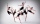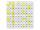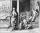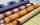# One hundred stamps

A hundred letter stamps cost a hundred crowns. Its costs are four levels - twenty tenths , one crown, two-crown and five-crown. How many are each type of stamps? How many does the problem have solutions?

Result

n =  66

#### Solution:

x1= 0.2*10 +1*85+2*4+5*1 = 100
x2= 0.2*15 +1*76+2*8+5*1 = 100
x3= 0.2*15 +1*79+2*4+5*2 = 100
x4= 0.2*20 +1*67+2*12+5*1 = 100
x5= 0.2*20 +1*70+2*8+5*2 = 100
x6= 0.2*20 +1*73+2*4+5*3 = 100
x7= 0.2*25 +1*58+2*16+5*1 = 100
x8= 0.2*25 +1*61+2*12+5*2 = 100
x9= 0.2*25 +1*64+2*8+5*3 = 100
x10= 0.2*25 +1*67+2*4+5*4 = 100
x11= 0.2*30 +1*49+2*20+5*1 = 100
x12= 0.2*30 +1*52+2*16+5*2 = 100
x13= 0.2*30 +1*55+2*12+5*3 = 100
x14= 0.2*30 +1*58+2*8+5*4 = 100
x15= 0.2*30 +1*61+2*4+5*5 = 100
x16= 0.2*35 +1*40+2*24+5*1 = 100
x17= 0.2*35 +1*43+2*20+5*2 = 100
x18= 0.2*35 +1*46+2*16+5*3 = 100
x19= 0.2*35 +1*49+2*12+5*4 = 100
x20= 0.2*35 +1*52+2*8+5*5 = 100
x21= 0.2*35 +1*55+2*4+5*6 = 100
x22= 0.2*40 +1*34+2*24+5*2 = 100
x23= 0.2*40 +1*37+2*20+5*3 = 100
x24= 0.2*40 +1*40+2*16+5*4 = 100
x25= 0.2*40 +1*43+2*12+5*5 = 100
x26= 0.2*40 +1*46+2*8+5*6 = 100
x27= 0.2*40 +1*49+2*4+5*7 = 100
x28= 0.2*45 +1*28+2*24+5*3 = 100
x29= 0.2*45 +1*31+2*20+5*4 = 100
x30= 0.2*45 +1*34+2*16+5*5 = 100
x31= 0.2*45 +1*37+2*12+5*6 = 100
x32= 0.2*45 +1*40+2*8+5*7 = 100
x33= 0.2*45 +1*43+2*4+5*8 = 100
x34= 0.2*50 +1*22+2*24+5*4 = 100
x35= 0.2*50 +1*25+2*20+5*5 = 100
x36= 0.2*50 +1*28+2*16+5*6 = 100
x37= 0.2*50 +1*31+2*12+5*7 = 100
x38= 0.2*50 +1*34+2*8+5*8 = 100
x39= 0.2*50 +1*37+2*4+5*9 = 100
x40= 0.2*55 +1*16+2*24+5*5 = 100
x41= 0.2*55 +1*19+2*20+5*6 = 100
x42= 0.2*55 +1*22+2*16+5*7 = 100
x43= 0.2*55 +1*25+2*12+5*8 = 100
x44= 0.2*55 +1*28+2*8+5*9 = 100
x45= 0.2*55 +1*31+2*4+5*10 = 100
x46= 0.2*60 +1*10+2*24+5*6 = 100
x47= 0.2*60 +1*13+2*20+5*7 = 100
x48= 0.2*60 +1*16+2*16+5*8 = 100
x49= 0.2*60 +1*19+2*12+5*9 = 100
x50= 0.2*60 +1*22+2*8+5*10 = 100
x51= 0.2*60 +1*25+2*4+5*11 = 100
x52= 0.2*65 +1*4+2*24+5*7 = 100
x53= 0.2*65 +1*7+2*20+5*8 = 100
x54= 0.2*65 +1*10+2*16+5*9 = 100
x55= 0.2*65 +1*13+2*12+5*10 = 100
x56= 0.2*65 +1*16+2*8+5*11 = 100
x57= 0.2*65 +1*19+2*4+5*12 = 100
x58= 0.2*70 +1*1+2*20+5*9 = 100
x59= 0.2*70 +1*4+2*16+5*10 = 100
x60= 0.2*70 +1*7+2*12+5*11 = 100
x61= 0.2*70 +1*10+2*8+5*12 = 100
x62= 0.2*70 +1*13+2*4+5*13 = 100
x63= 0.2*75 +1*1+2*12+5*12 = 100
x64= 0.2*75 +1*4+2*8+5*13 = 100
x65= 0.2*75 +1*7+2*4+5*14 = 100
x66= 0.2*80 +1*1+2*4+5*15 = 100

Leave us a comment of example and its solution (i.e. if it is still somewhat unclear...):

Showing 0 comments:Be the first to comment!#### To solve this example are needed these knowledge from mathematics:

Do you solve Diofant problems and looking for a calculator of Diofant integer equations?

## Next similar examples:

1. Dance ensembleThe dance ensemble took the stage in pairs. During dancing, the dancers gradually formed groups of four, six and nine. How many dancers have an ensemble?
2. Toy carsPavel has a collection of toy cars. He wanted to regroup them. But in the division of three, four, six, and eight, he was always one left. Only when he formed groups of seven, he divided everyone. How many toy cars have in the collection?
3. Two-digit numberI am a two-digit number less than 20. When I divided by three, then you get the rest 1 and when you divide me by four you get also rest 1. What number am I?
4. Six-digit primesFind all six-digit prime numbers that contain each one of digits 1,2,4,5,7 and 8 just once. How many are they?
5. Year 2018The product of the three positive numbers is 2018. What are the numbers?
6. Primes 2Which prime numbers is number 2025 divisible?
7. ClassWhen Pythagoras asked how many students attend the school, said: "Half of the students studying mathematics, 1/4 music, seventh silent and there are three girls at school." How many students had Pythagoras at school?
8. Digit sumDetermine for how many integers greater than 900 and less than 1,001 has digit sum digit of the digit sum number 1.
9. Divisibility 2How many divisors has integer number 13?
10. Sum of two primesChristian Goldbach, a mathematician, found out that every even number greater than 2 can be expressed as a sum of two prime numbers. Write or express 2018 as a sum of two prime numbers.
11. SchoolLess than 500 pupils attend school. When it is sorted into pairs, one pupil remains. Similarly, when sorted into 3, 4, 5 and 6 members team one remains. Sorted to seven members teams, no left behind. How many pupils are attending this school?
12. PackageThe package has no more than 67 m of cloth. If we just cut it all on the blouses or all on dresses, no cloth left remain. On the one blouse consumes 3.8 m of cloth and on one dress 1.7 m. Determine the amount of the cloth in the package.
13. Prime factorsWrite 98 as product of prime factors
14. LCM of two numberFind the smallest multiple of 63 and 147
15. Unknown numberUnknown number is divisible by exactly three different primes. When we compare these primes in ascending order, the following applies: • Difference first and second prime number is half the difference between the third and second prime numbers. • The produ
16. TogetherIf 8 men, 10 women, 16 children collects ₹1024 in 4 days, how many days will be required for 6 men, 5 women and 4 boys to collect ₹768? (₹ is Indian Rupee)
17. Z9–I–4 MO 2017Numbers 1, 2, 3, 4, 5, 6, 7, 8 and 9 were prepared for a train journey with three wagons. They wanted to sit out so that three numbers were seated in each carriage and the largest of each of the three was equal to the sum of the remaining two. The conducto